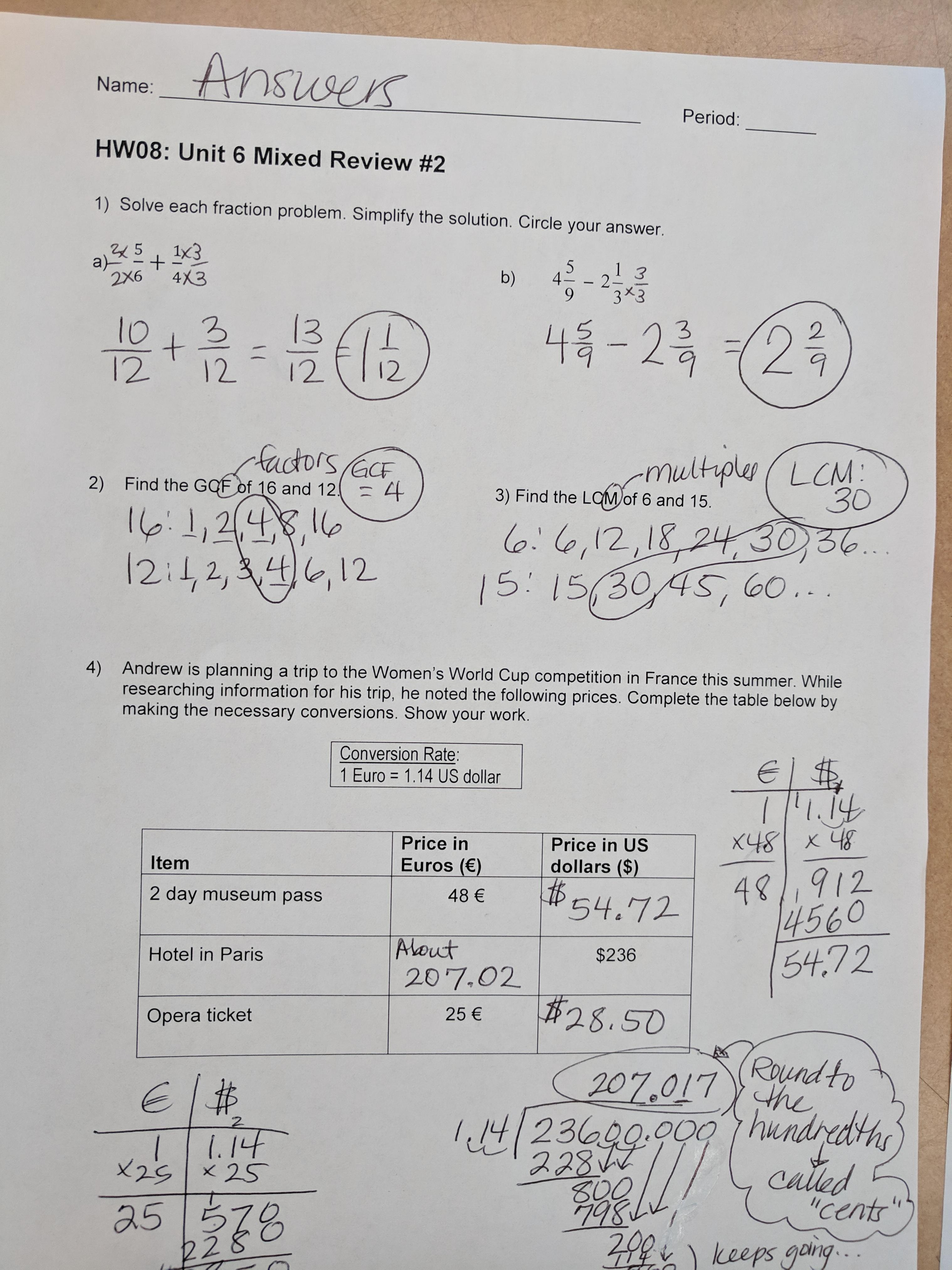buzzminer.co

Intro to Percents - Level 1 This four page worksheet introduces percents. It relates percents to one hundred and fractions. It also uses shading to help students visualize percentages and covers numbers that can not be separated into 100 using the four basic percents (25%, 50%, 75%, 100%). You could use it like this, Ninety-seven percent of my shirts are too small. According to recent data, Coca-Cola owns greater than 17 percent of the soft drink market, followed by Diet Coke at nearly ten percent, and trailed by Pepsi, at just under nine percent. Percents and Decimals - All Levels This one page worksheet has students write the percent as a decimal and write the decimal as a percent. Key concept: A percent is out of 100, which means you need to always move the decimal two places. Percent to decimal you move two spaces to the left, and decimal to percent you move two spaces to the right.

i-time

Studying for the post-formative. Students should be able to explain and use ratios and proportions to answer the pre-formative questions: 1-12 & 17-20

Math: Practice Solving Proportions

Classroom Activity:

• Checking the back of the Percent Worksheet
• Kahoot

Homework: Due Monday

Unit Rate Ratios

• Student Choice: Set up the proportion for all problems
• Percent Word Problems Student chooses 6 problems (combination of finding the percent, part, or whole)
• Percent Calculation Student completes 4 problems on each page

Math: Solving Percent Problems using the different strategies to solve proportions: 1) Between; 2)Within; 3) Simplifying; 4) Cross Products

Classroom Activity:

• Finishing up using Proportion Solving Strategies to solve percent problems: Percent Worksheet
• Working with a partner on Percent Worksheet, and BuzzMath
• Practice assignment: Student chooses worksheet to show understanding of working with percents.
• Percent Word Problems Student chooses 6 problems (combination of finding the percent, part, or whole)
• Percent Calculation Student completes 4 problems on each pageHomework: Due Monday

• Student choice Practiced Worksheets
• Study Pre-formative

Math: Solving Percent Problems using the different strategies to solve proportions: 1) Between; 2)Within; 3) Simplifying; 4) Cross Products

Classroom Activity:

• Review Strategies to solve proportions Percent Worksheet (p.1)
• Using Proportion Solving Strategies to solve percent problems: Percent Worksheet
• Working with a partner on Percent Worksheet, and BuzzMath

Homework: Due Thursday

• Back of the Percent Worksheet
• BuzzMath- Challenge 2: Finding Percent of a Quantity
• Pre-formative questions: 1-12 & 17-20Math: Solving Percent Problems using the different strategies to solve proportions: 1) Between; 2)Within; & 3) Simplifying

Classroom Activity:• Review Strategies to solve proportions Proportion Solving Strategies
• Using Proportion Solving Strategies to solve percent problems: Percent Worksheet

Homework: Due Thursday

• Back of the Percent Worksheet
• BuzzMath- Challenge 2: Finding Percent of a Quantity
• Pre-formative questions: 1-12 & 17-20

Ratios And Percents Worksheets

Math: Solve problems of percents by setting up a proportion (equivalent ratios) to justify their answer.

Classroom Activity:

Homework:

Ratio And Unit Rates Practice

• BuzzMath- Challenge 2: Finding Percent of a Quantity
• Pre-formative questions: 1-12 & 17-20
Coments are closed
Scroll to top